# Rigging Mechanics

Relevant to Blender v2.31

Armatures are great also for rigging mechanical stuff, like robots, WarriorMechs etc. (Figure 55).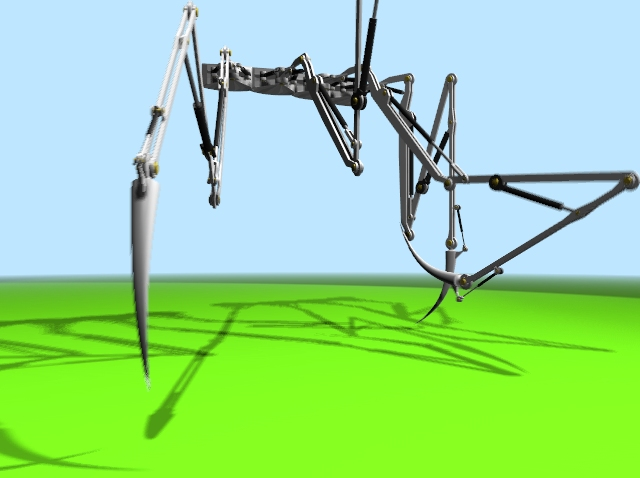Figure 55. Four spider-mech legs.

First step is to create the mesh for the arms. We are not here for organic, we are here for mechanics. So no single mesh thing. The arm/leg/whatever is made of rigid parts, each part is a single mesh, parts moves/rotates one with respect to the other.

Although Figure 55 has four spider-like legs arms, each of which have 5 sections, it is clearer to explain the tricks with just a single joint arm.

My suggestion is this: make the arm with two equal sections, and the forearm, on the right, made by just one section. Note the cylinders which represents the shoulder (left) the elbow (centre) and the wrist (right) (Figure 56).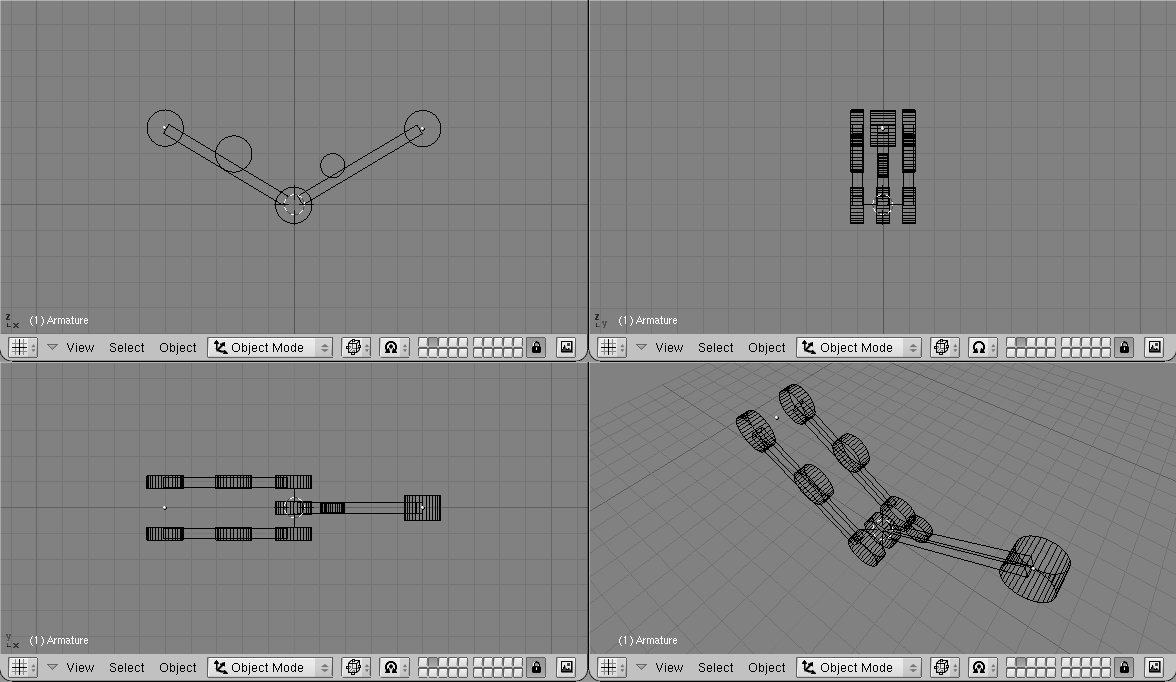Figure 56. The Arm model

The other cylinders in the middle of the arm and forearm are the places where the piston will be linked to.

Note that it is much easier if the axis of mutual rotation (shoulder, elbow, etc.) are exactly on grid points. This is not necessary though, if you master well Blender Snap menu.

## Pivot axis

Then add the mechanical axes in the pivot points. Theoretically you should add one at each joint and two for every piston. For the sake of simplicity here there are only the two axes for the piston, made with plain cylinders (Figure 57).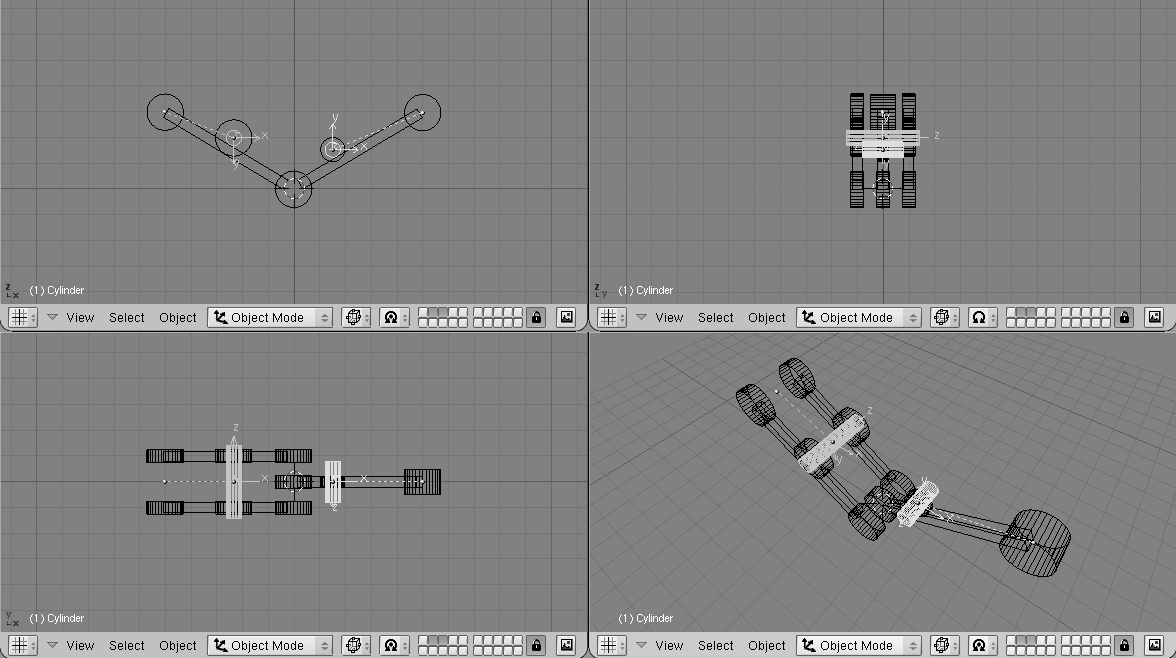Figure 57. The Arm model with pivot axis.

Note two things:

• It is fundamental that the centre of the mesh is exactly in the middle and exactly on the axis of rotation of the piston.

• Each axis must be parented to the pertinent arm mesh.

## The Armature

Now it is time to set up the armature. Just two bones are enough (Figure 58).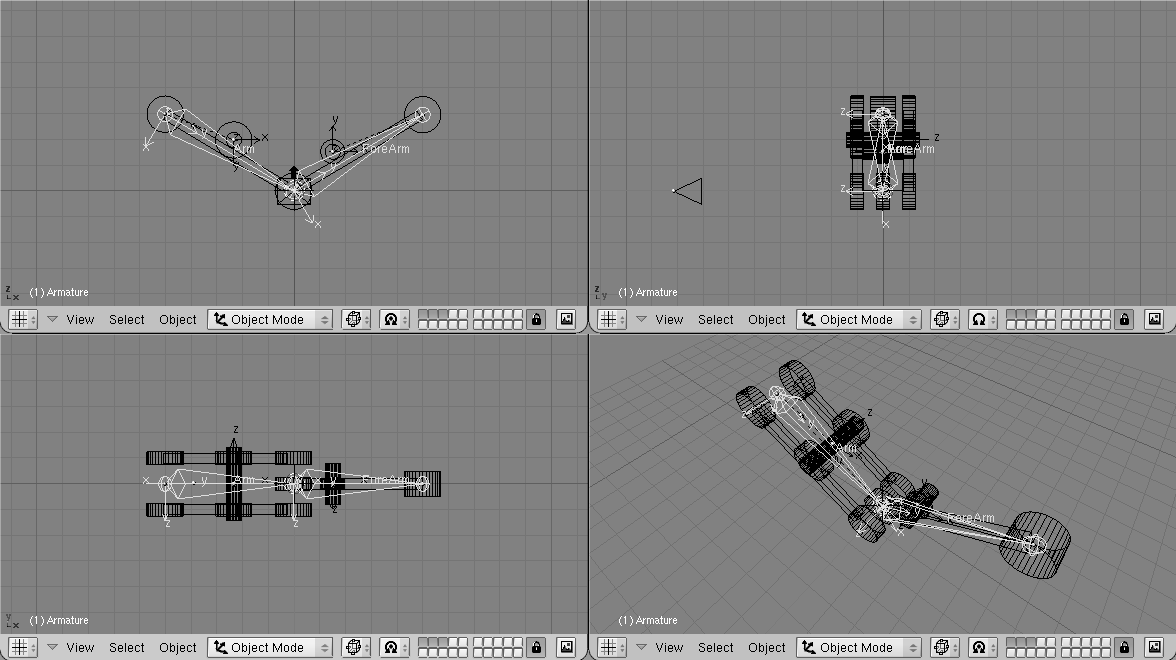Figure 58. The Arm model and its armature

To have an accurate movement, the joints must be precisely set on the pivoting axis (this is why I told you to place such axes on grid points before, so that you can use the Move Selected To Grid feature).

Name the bones smartly (Arm and Forearm, for example). Parent the Arm Mesh to the armature, selecting the Bone option and the Arm bone. Do the same with the forearm mesh and forearm bone.

 Parent to BoneParent to Bone effectively makes the Object follow the bone without any deformation. This is what should happen for a robot which is made by undeformable pieces of steel!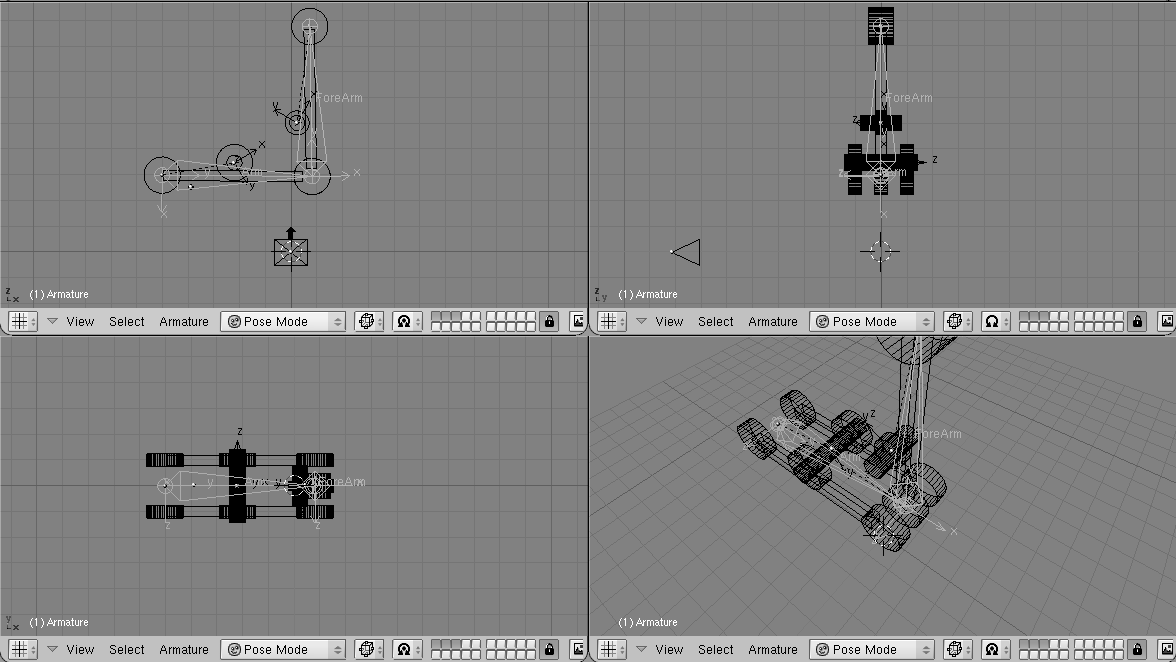Figure 59. The Arm model in Pose Mode

If you switch to Pose Mode you can move your arm by rotating the bones. (Figure 59). You can add an Inverse Kinematics (IK) solver as we did in the previous section if you like.

## Hydraulics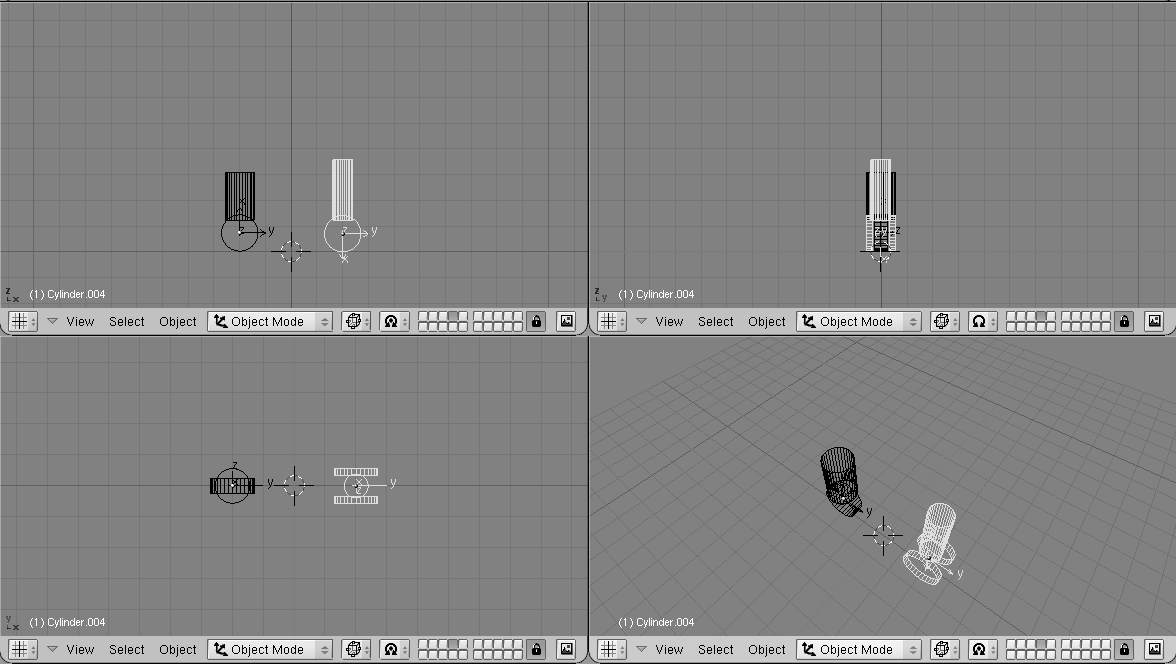Figure 60. Hydraulic piston.

Make a piston with two cylinders, a larger one and a thinner one, with some sort of nice head for linking to the pivoting points (Figure 60).

It is mandatory for the two pieces to have the mesh centre exactly on the respective pivoting axis.

Place them in the correct position and parent each piston piece to the pertinent mesh representing the axis. (Figure 61).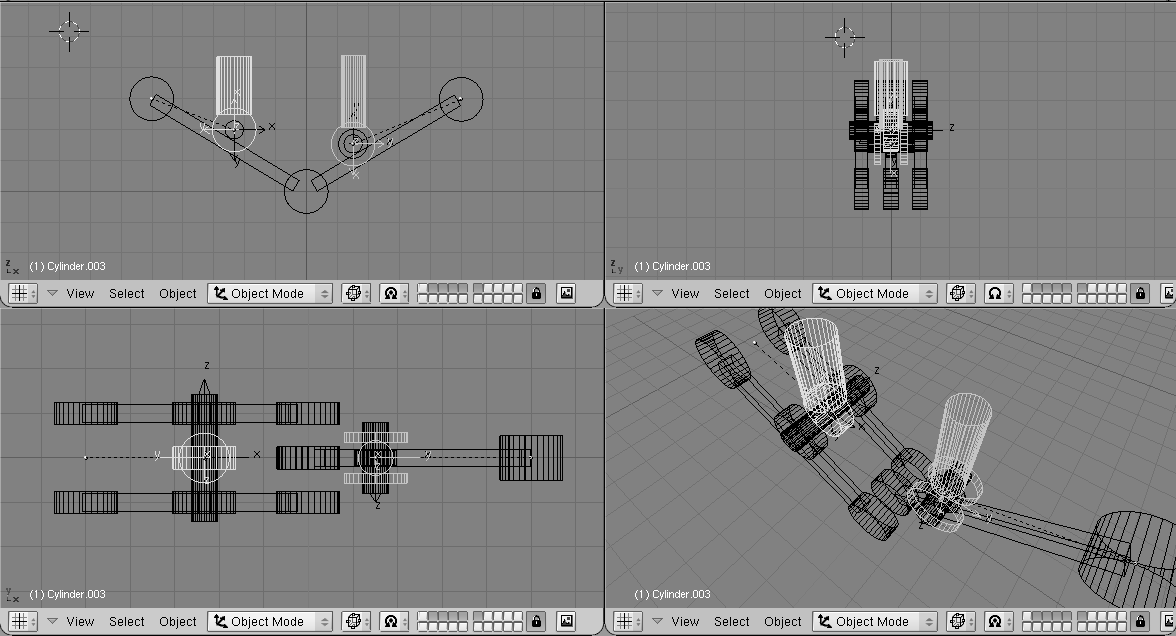Figure 61. Hydraulic piston on the arm.

If you now rotate the two pieces in the position they should have to form a correct still image you get a nice piston. (Figure 62, left).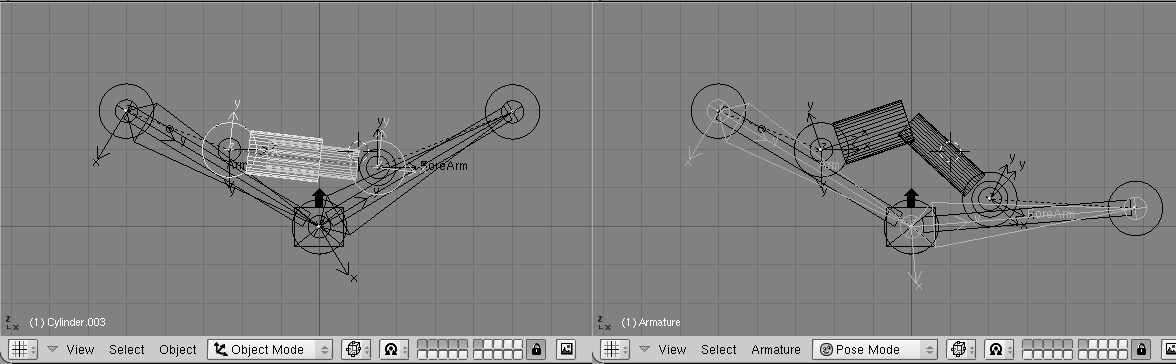Figure 62. Hydraulic piston in Pose Mode.

But if you switch to Pose Mode and start moving the Arm/Forearm the piston gets screwed up... (Figure 62, right).

To make a working piston you must make each half piston track the other half piston's pivot axis cylinder mesh (Not the other half piston! This would create a constraint loop). This is why the position of all the mesh centres is so critical (Figure 63).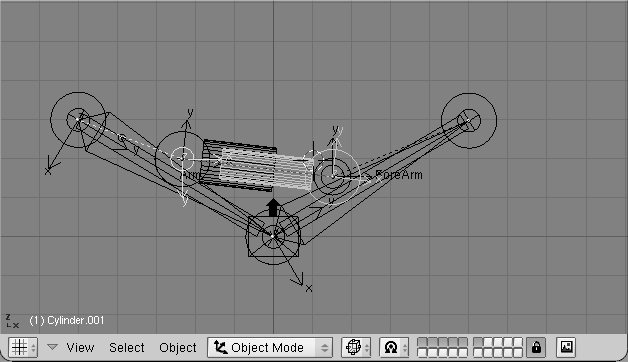Figure 63. Hydraulic piston with mutual tracking.

Select half a piston, select the other half piston's axis mesh, and, in Object Context (F7) and Constraints panel add a Track To Constraint. The buttons below X, Y... must be appropriately set (Figure 64).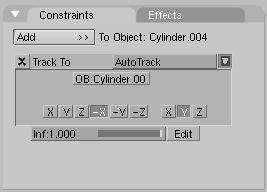Figure 64. Track settings.If you prefer Old Track, remember also to press the PowerTrack button in the Anim Setting Panel for a nicer result.

Now, if you switch to Pose Mode and rotate your bones the piston will extend and contract nicely, as it should in reality. (Figure 65).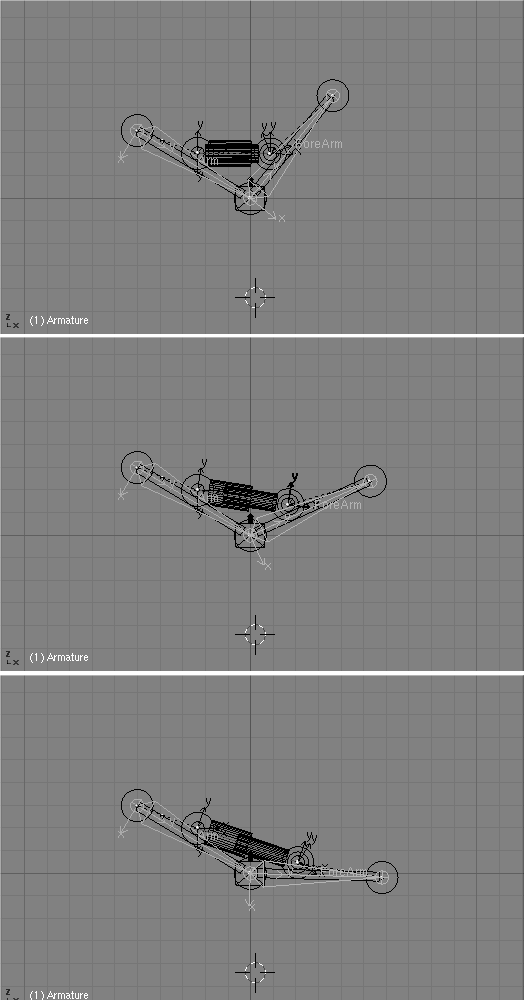Figure 65. Pose Mode for the arm with hydraulics.

The next issue is, since pistons work when pressurised oil is pumped into them, for a really accurate model we should add some hydraulic hoses. But how to place a nicely deforming tube going from arm to piston? The two ends should stick to two rigid bodies reciprocally rotating. This requires IK!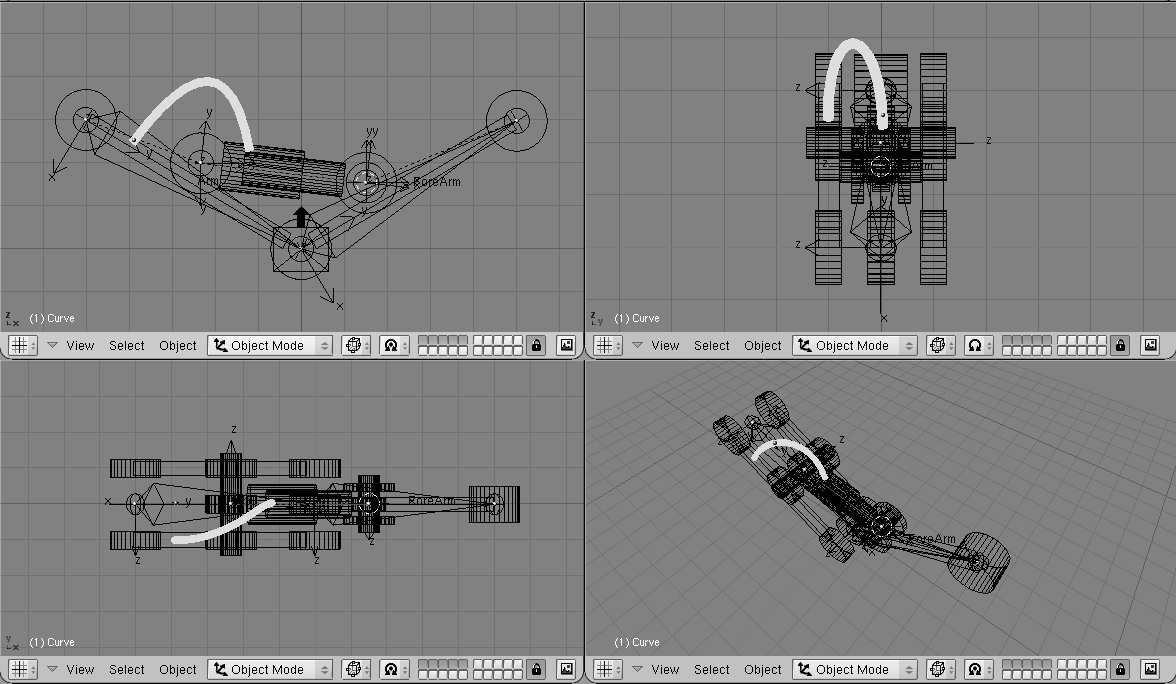Figure 66. Adding a flexible tube.

First add a mesh in the shape of the tube you want to model (Figure 66).

Personally I prefer to draw the tube in its bent position as a bevelled curve. This is done by adding a Bézier curve, adding a Bézier circle, and using the Bézier circle as BevOb of the Bézier curve. Then convert that to a mesh (ALT-C) to be able to deform it with an armature.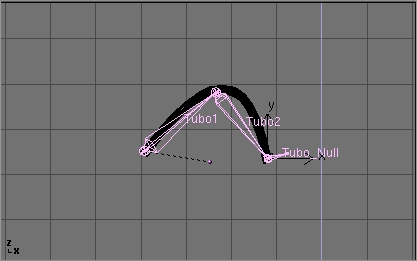Figure 67. Adding the armature to the tube.

Then add an armature. A couple of bones are enough. This armature should go from the tube's 'fixed' end to the tube's 'mobile' end. Add a third bone which will be used for the Inverse Kinematics solution (Figure 67).

Be sure that the armature is parented to the object where the 'fixed' part of the tube is, well, fixed. In this case the robot arm. Also add an Empty at the 'mobile' end of the tube. (Figure 68).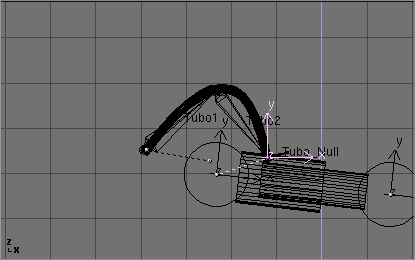Figure 68. The Empty for the IK Animation solution.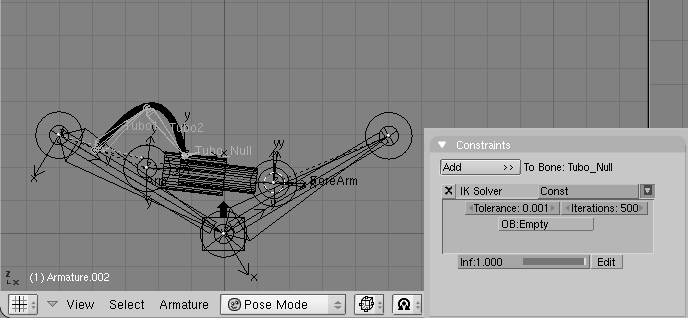Figure 69. IK constraint.

Parent the Empty to the 'mobile' part of the structure. In this case the outer part of the piston to which the tube is linked. In Pose Mode go to the Object Context and Constraints Panel. Select the last bone, the one which starts from where the tube ends, and Add a constraint. Select the IK solver type of constraint and select the newly created Empty as target Object OB:. (Figure 69). You can play with Tolerance: and Iterations: if you like.

Lastly, parent the tube to the Armature via the 'Armature' option. Create Vertex groups if you like. Now if, in Pose Mode, you move the arm, the two parts of the piston keep moving appropriately, and the Empty follows. This obliges the IK Armature of the tube to move, to follow the Empty, and, consequently, the Tube to deform (Figure 69).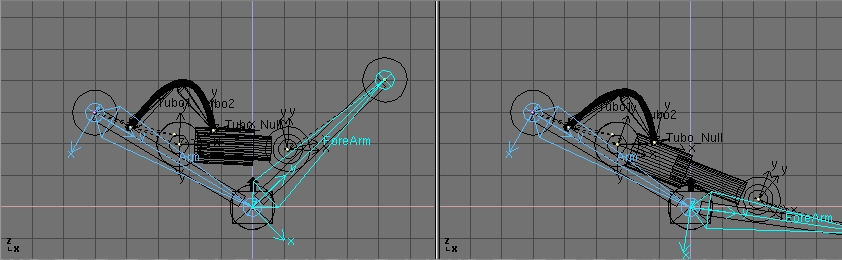Figure 70. Full robot arm in Pose Mode.You can use a bone of the Armature, instead of an Empty, as an IK solver, but in this case you cannot parent the bone to the moving object. You can on the other hand, use a Copy Location constraint, but this is not as easy since the copy location would move the end of the armature to the center of the moving object, which is not the right place.